# Wave front

(diff) ← Older revision | Latest revision (diff) | Newer revision → (diff)

wave front set, of a generalized function (distribution) or hyperfunction

A conical set in the cotangent bundle to the manifold on which the generalized function or hyperfunction in question is given, which characterizes its singularities. A hyperfunction is a sum of formal boundary values of holomorphic functions. Two such sums are identified if they are equivalent in the sense of equivalence given by an analogue of Bogolyubov's "edge-of-the-wedge" theorem (cf. Bogolyubov theorem), in which, however, one in no sense assumes that the holomorphic functions in question have limits.

The wave front set of a hyperfunction is also often called the analytic wave front set or the singular support (the last term is more often used in a completely-different sense, when it denotes the complement to the set of some sort of regularity of the generalized function on the manifold itself, and not in the cotangent bundle). The concept of the wave front set lies behind micro-local analysis, which is a complex of ideas and methods using wave front sets and other related concepts and techniques (in particular, pseudo-differential operators and Fourier integral operators) for studying partial differential equations (mainly linear equations).

Letbe a domain inand let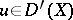, that is,is a generalized function on. Then the wave front set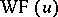ofis the closed conical subset of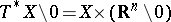defined as follows: If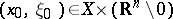, then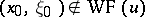means that there is a function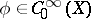, equal toin a neighbourhood of, and a conical neighbourhoodof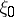in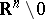, such that for every,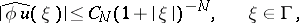wherethat is,is the Fourier transform of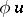.

Ifis a manifold andis a generalized function on(or, more generally, a generalized section of a smooth vector bundle), thenis defined in the same way as above (after transition to local coordinates). In this caseturns out to be a well-defined conical subset of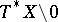(the cotangent bundle without the zero section).

One introduces the canonical projection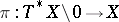. Then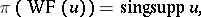(1)

where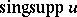is the complement of the largest open subset ofon whichcoincides with an infinitely-differentiable function. This relationship shows thatis actually a finer characteristic of the singularities ofthan.

Letbe a pseudo-differential operator of orderonwith principal symbol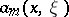, and let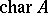be the set of its characteristic directions, that is,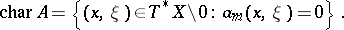Then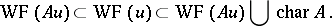(2)

Here the first inclusion characterizes the pseudo-locality of, and the second is a far-reaching generalization of the theorem on the smoothness of solutions of elliptic equations with smooth coefficients.

If the principal symbolofis real-valued, then the following theorem on the propagation of singularities holds: If one is given a connected pieceof a bicharacteristic (that is, a trajectory of the Hamiltonian vector field onwith Hamiltonian) that does not intersect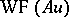, then either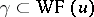or.

This theorem shows that the singularities of the solutions (that is, their wave front sets) of an equation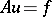with a smooth right-hand sidepropagate along the bicharacteristics of the principal symbol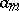of(see , , , , , ).

The analytic wave front setfor a generalized functioncan be defined in one of the following three equivalent (see ) ways (here, for simplicity,is a domain in):

1)if there are a neighbourhoodof, open proper convex conesinand functions, holomorphic in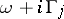, such that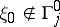,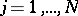, and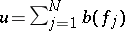, where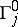is the cone dual to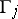and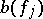is the boundary value of the holomorphic functionfor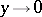,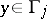, understood in the sense of weak convergence of generalized functions. This definition is also applicable to hyperfunctions if the boundary value is interpreted differently.

2) Let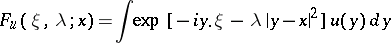(a generalized Fourier transform); thenif and only if for any functionthat is analytic in a neighbourhood ofthere are a conical neighbourhoodofand positive constantssuch that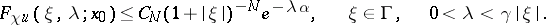3)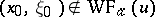if and only if there are a neighbourhoodofin, a bounded sequence of generalized functions,with compact support, and a constant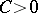, such that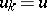inand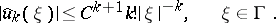There is an analogue of the property (1) for the analytic wave front:whereis the complement of the largest set on whichis real-analytic. There is an analogue of the property (2), where one can take fora differential operator with real-analytic coefficients or an analytic pseudo-differential operator (see , , , , ). For such an operatorwith a real principal symbol, a theorem on the propagation of the analytic wave front set holds, analogous to the theorem stated above for the ordinary wave front set (see ).

How to Cite This Entry:
Wave front. Encyclopedia of Mathematics. URL: http://encyclopediaofmath.org/index.php?title=Wave_front&oldid=18893
This article was adapted from an original article by M.A. Shubin (originator), which appeared in Encyclopedia of Mathematics - ISBN 1402006098. See original article